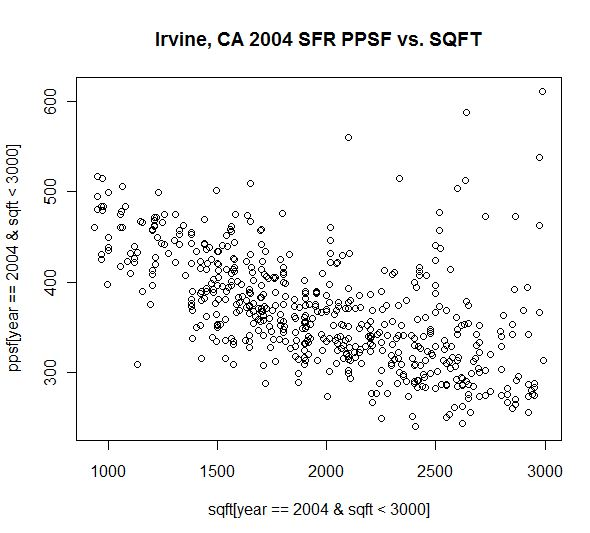Why the Price Per Square Foot (PPSF) Metric Creates a Distorted Trend

Price per square foot, like the median, is simply another summary measure for the dataset involving home sale prices. Neither of these measures is inherently flawed as a standalone value. However, when we use either of them to compare month-over-month trends, we introduce distortion due to the shift in the mix of the underlying properties. The problems with using the median home value as a trend indicator were discussed here.

In many parts of the country, the value of a single family home is a function of both the significant value of the underlying land and the value of the structure built upon such land. Because the value of the underlying land is fixed (and not inherently related to the value of the structure built on the land), it stands to reason that doubling the size of a home will not double the value of such a home. If a small home is worth \$V in the market, that \$V decomposes into \$VL and \$VS, where \$VL is the value of the land and \$VS is the value of the structure.

Though it is not necessarily the case, let's suppose the doubling the size of the structure doubled the value of the structure. Thus, a home that's twice as large as our small home would now be worth \$XL + \$2XS. Because the land component of this relationship is fixed, the ratio of the larger home's value to the smaller home's value is (VL + 2VS)/(VL + VS). If the land were worth zero (VL=0), that ratio simplifies into 2VS/VS = 2. However, since land is typically worth much more than zero, we can prove that this ratio is less than 2. In fact, the greater the percentage of value represented by the land, the smaller the percentage increase in the value of a property when the structure is enlarged.

With the above result in mind, it mathematically follows that if the home value increases at a slower rate than rate of increase in the size increase of the structure, then the price per square foot must *decrease* as size increases.

We can also empirically provide evidence for this mathematical result by looking at actual sales from our Irvine, CA case study dataset.The above scatterplot shows the relationship between the price per square foot (y-axis) and the sqft (x-axis) for true detached single family residences of less than 3000 square feet in Irvine, CA in the year 2004. It's clear to see that as sqft increases, we see a corresponding decrease in price per square foot. If we use a simple linear regression to check the validity of this relationship, we find that R-squared is 0.32 with a P-value of 2e-16. For non-statisticians out there, this result indicates that 32% of the change in price per square foot is explained by the change in the size of the property and that such a relationship exists with near certainty (as opposed to by fluke or chance).

The above analysis and graph was created using the excellent open source (and free) statistical analysis package called R. If you have more than a passing interest in data analytics, statistics, data mining, or mathematics, we highly recommend investigating whether R might be a valuable tool for you. It has just as much algorithmic power as its major commercial competitors, such as SAS and SPSS (which are also fine tools themselves if your budget is larger). R does lack a user-friendly interface and may stumble with very large datasets, but is an excellent tool for most statistical purposes.

Because the price per square foot is affected based on the size of what is selling, it's clear (both theoretically and empirically) that using the price per square foot as a trend indicator will create distortions in the short term. Interestingly, those distortions will tend to move in the opposite direction as the distortions created by the median. As larger homes make up a greater portion of the mix of sales, the median will increase. However, the price per square foot will decrease. Analysts who are particularly enthralled with either of these summary metrics might be well advised to use both each month to evaluate trends in the market.

Next, what about the Case-Shiller index?